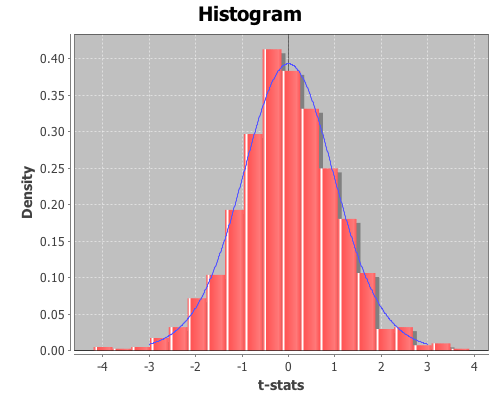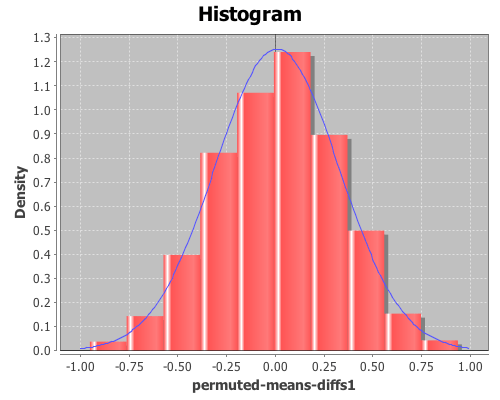# Significance testing with randomization

We can use randomization (a.k.a. permutation tests) for significance testing by comparing a statistic calculated from a sample with the distribution of statistics calculated from samples where the null hypothesis is true.

In an earlier post, I demonstrated how to perform a t-test in Incanter. The p-value calculated for the test is based on the assumption that the distribution of test statistics when the null hypothesis, that the two sample means are identical, is true is a t-distribution. Let’s see if that assumption appears correct by generating 1000 samples of data where the null hypothesis is true, calculating the t-statistic for each, and then examining the distribution of statistics.

We can then compare the original t-statistic with this distribution and determine the probability of seeing that particular value when the null hypothesis is true. If the probability is below some cutoff (typically, 0.05) we reject the null hypothesis.

``(use '(incanter core stats datasets charts))``

``(def data (to-matrix (get-dataset :plant-growth)))``

Break the first column of the data into groups based on treatment type (second column) using the group-by function,

``(def groups (group-by data 1 :cols 0))``

The first group is the the control, the other two are treatments. View box-plots of the three groups, using the :group-by option of the box-plot function,

``````(view (box-plot (sel plant-growth :cols 0)
:group-by (sel plant-growth :cols 1)))``````and then view the means of the three groups

``(map mean groups)``

This returns (5.032 4.661 5.526). So, the question is, are these means statistically different from each other?

Run the t-tests comparing the means of the first and second treatment groups with the control,

``````(def t1 (t-test (first groups) :y (second groups)))
(def t2 (t-test (first groups) :y (last groups)))``````

and view the test statistic and p-value for the first treatment,

``````(:t-stat t1)
(:p-value t1)``````

which are 1.191 and 0.2503, respectively, and the second treatment,

``````(:t-stat t2)
(:p-value t2)``````

which are -2.134 and 0.0479, respectively. These values provide evidence that the first treatment is not statistically significantly different from the control, but the second treatment is.

The above p-values are based on the assumption that the distribution of t-test statistics, when the null hypothesis is true, is a t-distribution. Let’s see if that assumption appears correct for these samples by generating 1000 samples where the null hypothesis is true. We can do that by permuting values between each of the treatments and the control using the sample-permutations function.

``````
(def perm-groups1 (sample-permutations 1000
(first groups)
(second groups)))

(def perm-groups2 (sample-permutations 1000
(first groups)
(last groups)))``````

Now create a function, based on t-test, that takes two sequence and returns just the :t-stat value

``(defn t-stat [x y] (:t-stat (t-test x :y y)))``

Calculate the t-test statistics for each of the permuted versions of the two treatments and control with the map function.

``````(def t-stats1 (map t-stat
(first perm-groups1)
(second perm-groups1)))

(def t-stats2 (map t-stat
(first perm-groups2)
(second perm-groups2)))``````

Now plot the distribution of the t-test statistics, and overlay the pdf of a t-distribution for the for the first treatment.

``````(doto (histogram t-stats1 :density true :nbins 20)
(add-function #(pdf-t % :df (:df t1)) -3 3)
view)``````The distribution looks like a Student’s t. Now inspect the mean, standard deviation, and 95% confidence interval for the distribution from the first treatment.

``(mean t-stats1)``

The mean is 0.023,

``(sd t-stats1)``

the standard deviation is 1.064,

``(quantile t-stats1 :probs [0.025 0.975])``

and the 95% confidence interval is (-2.116 2.002). Since the t-statistics for the original sample, 1.191, falls within the range of the confidence interval, we cannot reject the null hypothesis; the mean of the first treatment and the control are statistically indistinguishable.

Now view their mean, sd, and 95% confidence interval for the second treatment,

``(mean t-stats2)``

The mean is -0.014,

``(sd t-stats2)``

The standard deviation is 1.054,

``(quantile t-stats2 :probs [0.025 0.975])``

and the 95% confidence interval is (-2.075 2.122). In this case, the original t-statistic, -2.134, is outside of the confidence interval, therefore the null hypothesis should be rejected; the second treatment is statistically significantly different than the control.

In both cases the permutation tests agreed with the standard t-test p-values. However, permutation tests can be used to test significance on sample statistics that do not have well known distributions like the t-distribution.

Comparing means without the t-statistic

Using a permutation test, we can compare means without using the t-statistic at all. First, define a function to calculate the difference in means between two sequences.

``````
(defn means-diff [x y]
(minus (mean x) (mean y)))``````

Calculate the difference in sample means between the first treatment and the control.

``````
(def samp-mean-diff (means-diff (first groups)
(second groups))) ``````

This returns 0.371. Now calculate the difference of means of the 1000 permuted samples by mapping the means-diff function over the rows returned by the sample-permutations function we ran earlier.

``````
(def perm-means-diffs1 (map means-diff
(first perm-groups1)
(second perm-groups1)))``````

This returns a sequence of differences in the means of the two groups.

Plot a histogram of the perm-means-diffs1 using the density option, instead of the default frequency, and then add a pdf-normal curve with the mean and sd of perm-means-diffs data for a visual comparison.

``````
(doto (histogram perm-means-diffs1 :density true)
(pdf-normal (range -1 1 0.01)
:mean (mean perm-means-diffs1)
:sd (sd perm-means-diffs1)))
view)``````The permuted data looks normal. Now calculate the proportion of values that are greater than the original sample means-difference. Use the indicator function to generate a sequence of ones and zeros, where one indicates a value of the perm-means-diff sequence is greater than the original sample mean, and zero indicates a value that is less.

Then take the mean of this sequence, which gives the proportion of times that a value from the permuted sequences are more extreme than the original sample mean (i.e. the p-value).

``````(mean (indicator #(> % samp-mean-diff)
perm-means-diffs1))``````

This returns 0.088, therefore the calculated p-value is 0.088, meaning that 8.8% of the permuted sequences had a difference in means at least as large as the difference in the original sample means. Therefore there is no evidence (at an alpha level of 0.05) that the means of the control and treatment-one are statistically significantly different.

We can also test the significance by computing a 95% confidence interval for the permuted differences. If the original sample means-difference is outside of this range, there is evidence that the two means are statistically significantly different.

``(quantile perm-means-diffs1 :probs [0.025 0.975]) ``

This returns (-0.606 0.595), but since the original sample mean difference was 0.371, which is inside the interval, this is further evidence that treatment one’s mean is not statistically significantly different than the control.

Now, calculate the p-values for the difference in means between the control and treatment two.

``````
(def perm-means-diffs2 (map means-diff
(first perm-groups2)
(second perm-groups2)))

(def samp-mean-diff (means-diff (first groups)
(last groups)))

(mean (indicator #(< % samp-mean-diff)
perm-means-diffs2))

(quantile perm-means-diffs2
:probs [0.025 0.975])``````

The calculated p-value is 0.022, meaning that only 2.2% of the permuted sequences had a difference in means at least as large as the difference in the original sample means. Therefore there is evidence (at an alpha level of 0.05) that the means of the control and treatment-two are statistically significantly different.

The original sample mean difference between the control and treatment two was -0.4939, which is outside the range (-0.478 0.466) calculated above. This is further evidence that treatment two’s mean is statistically significantly different than the control.

The complete code for this example is here.